Inequalities on a Number Line

## Inequalities on a Number Line

Inequalities are used when a number is not equal to another value. An inequality will use one of the inequality signs:

< less than

≤ less than or equal to;

> greater than;

≥ greater than or equal to.

In algebra, the inequality will refer to a number, or range of numbers, which are either greater than, greater than or equal to, less than, or less than or equal to a fixed value.

This can be shown on a number line using lines and circles. The line indicates the range of possible values. The circle is the end point of the line.

If a circle is filled, then it is equal to or greater/less than the fixed value. If the circle is not filled, then the fixed value is not included.

a) x < 3; the circle is not filled so the value is less than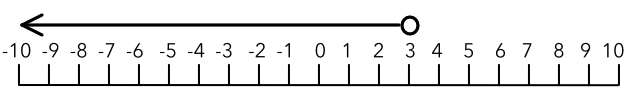b) x ≤ 3; the circle is filled, so the value is less than or equal to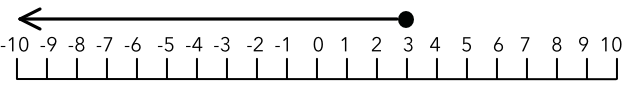Two inequalities can be applied to a value at the same time. On a number line, this would be represented by a line with a circle at each end.

c) -3 < x ≤ 5; -3 is not included (unfilled). 5 is included (filled)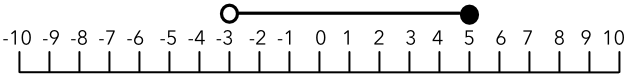## Example 1

Show, on a number line, the inequality x > 6.

The greater than sign indicates that the values must be greater than 6, therefore the circle must not be filled. The arrow indicates that all numbers greater than 6 are included.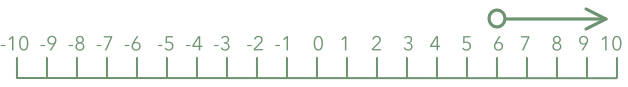## Example 2

What inequality is shown by the number line, below?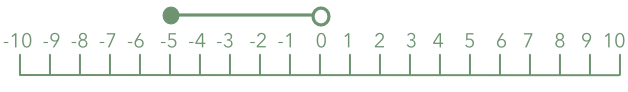x is greater than or equal to -5; and x is less than 0.

Note that the filled circle indicates equal to or greater than so -5 is included; and the circle that is not filled indicates less than so 0 is not included.

Answer: -5 ≤ x < 0